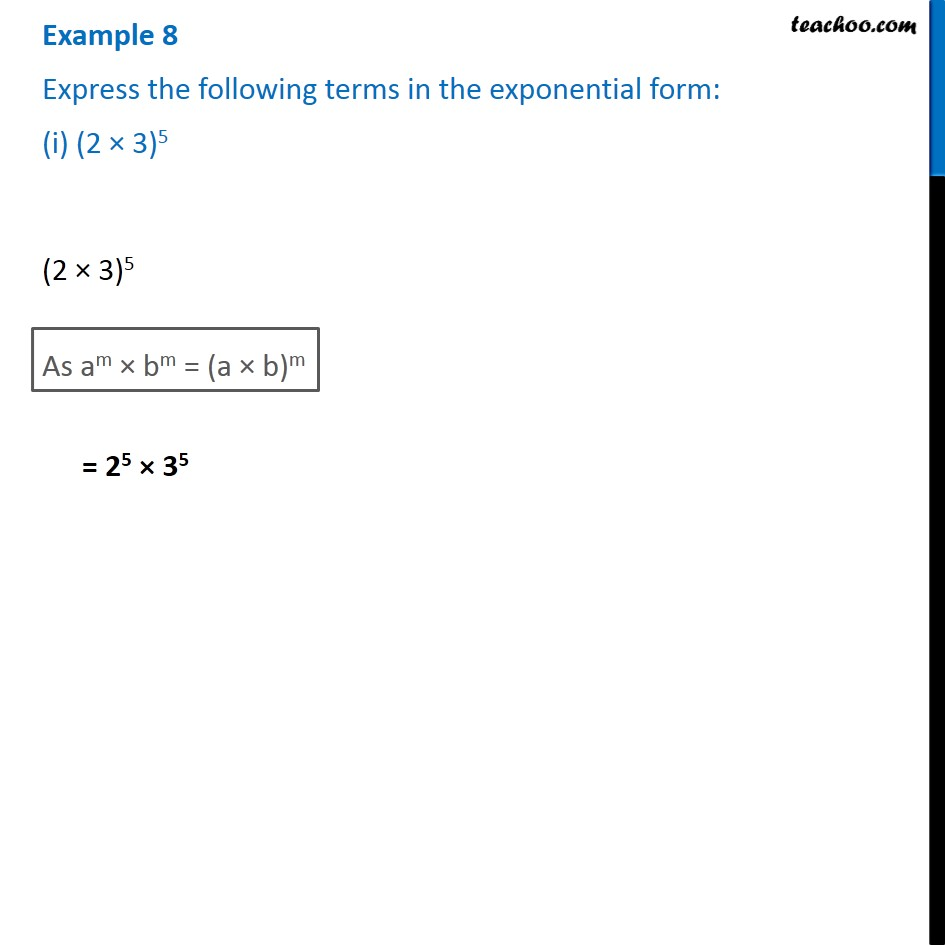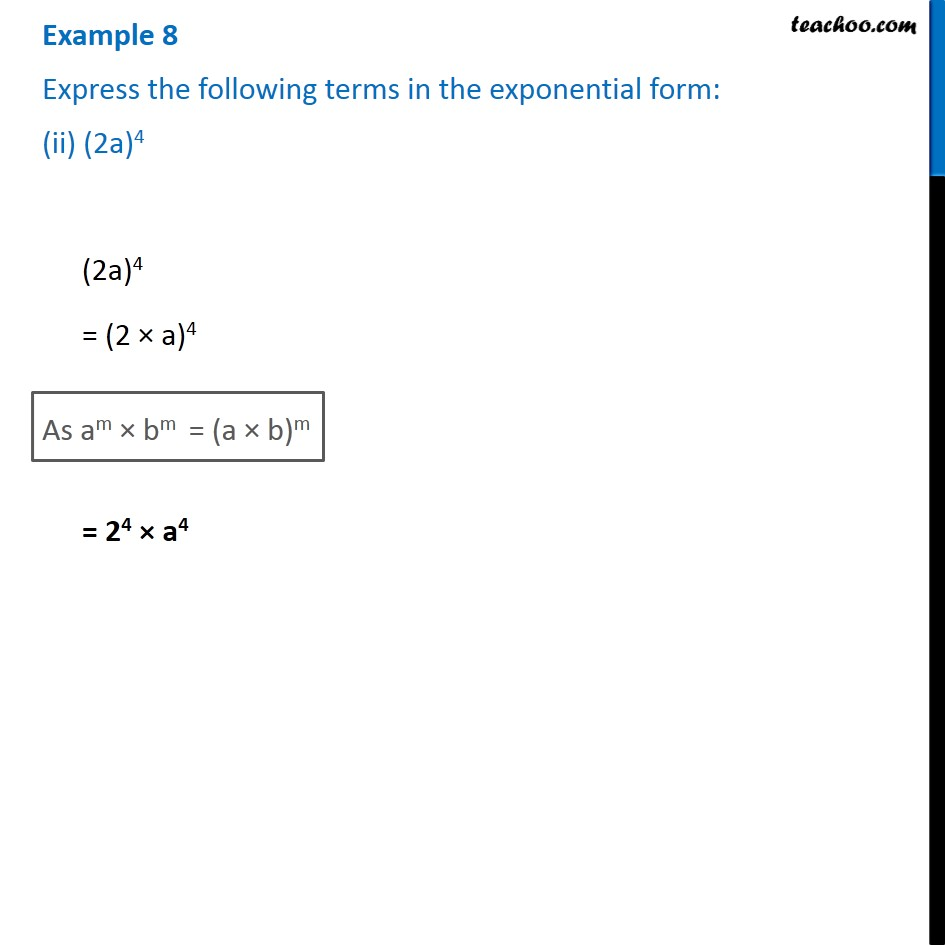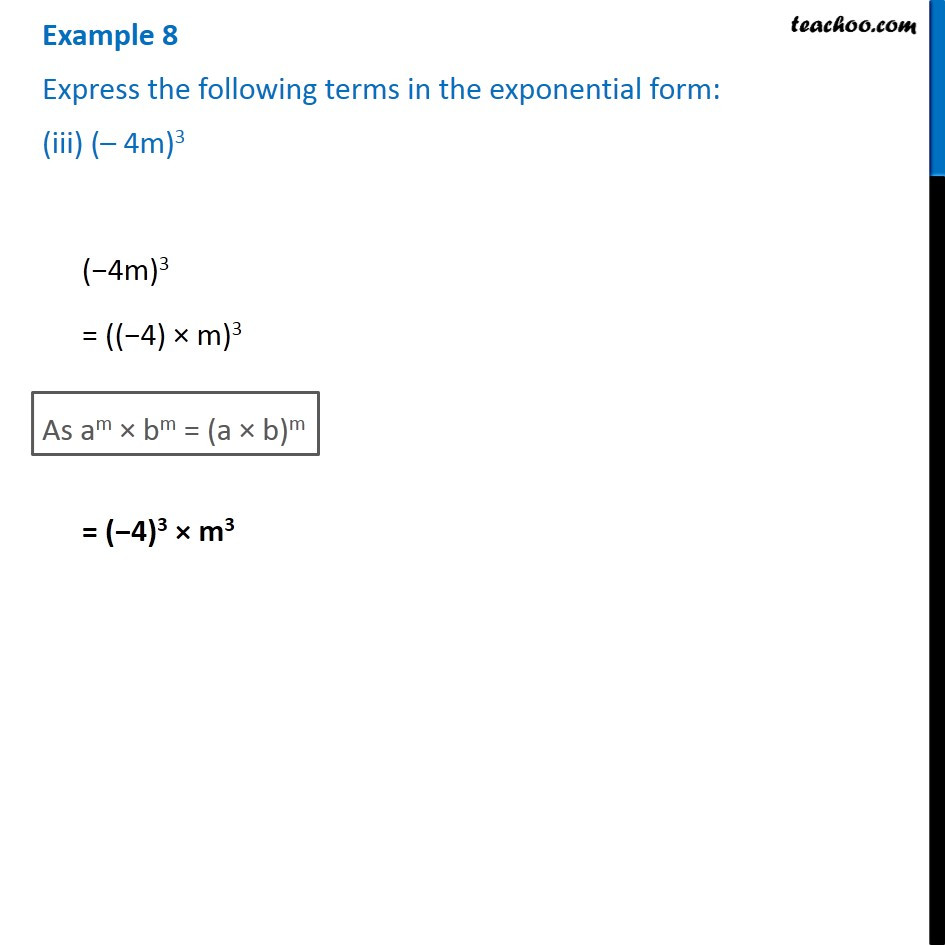1. Chapter 13 Class 7 Exponents and Powers
2. Serial order wise
3. Examples

Transcript

Example 8 Express the following terms in the exponential form: (i) (2 × 3)5 (2 × 3)5 As am × bm = (a × b)m = 25 × 35 Example 8 Express the following terms in the exponential form: (ii) (2a)4 (2a)4 = (2 × a)4 As am × bm = (a × b)m = 24 × a4 Example 8 Express the following terms in the exponential form: (iii) (– 4m)3 (−4m)3 = ((−4) × m)3 As am × bm = (a × b)m = (−4)3 × m3

Examples